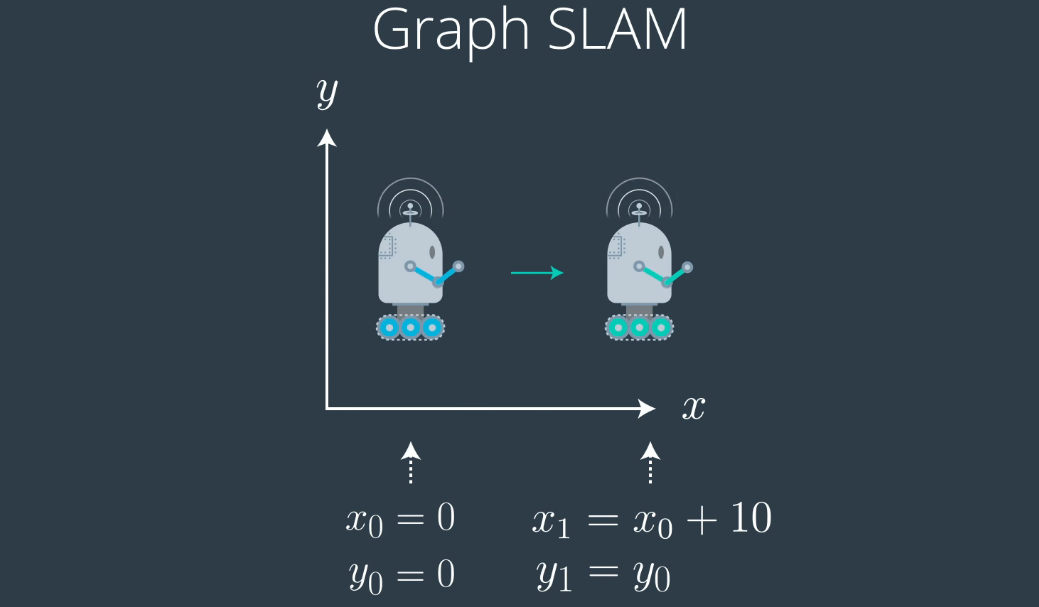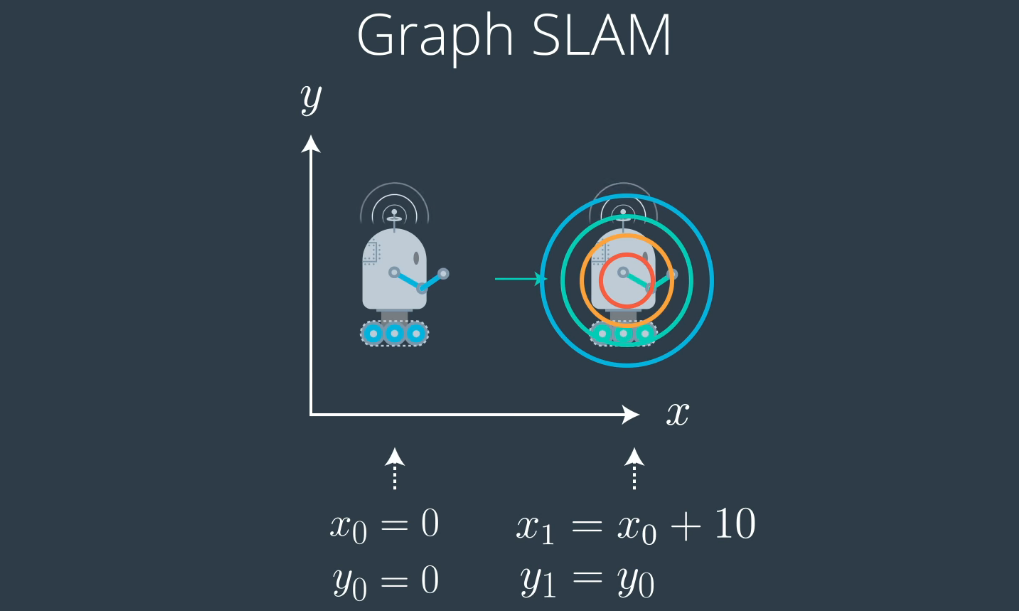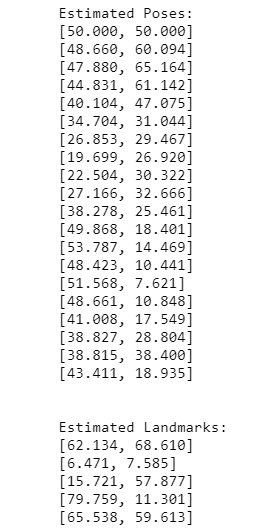# 图SLAM：Noob的同时本地化和映射指南### 什么是SLAM？

#### SLAM的工作：### 实作

#### SLAM输入：

• N：机器人将要移动和感应的时间步数。
• num_landmarks：世界上的地标数量。
• world_size：你的世界的大小（w / h）。
• motion_noise：与运动相关的噪声；运动的更新置信度应为1.0/motion_noise.
• measurement_noise：与测量/传感相关的噪声；测量的更新权重应为1.0/measurement_noise.
import numpy as np
from helpers import make_data

#slam的实现应该使用以下输入

#请随意更改这些输入值并查看其响应方式！

# 世界参数
num_landmarks      = 5        # number of landmarks
N                  = 20       # time steps
world_size         = 100.0    # size of world (square)

# 机器人参数
measurement_range  = 50.0     # range at which we can sense landmarks
motion_noise       = 2.0      # noise in robot motion
measurement_noise  = 2.0      # noise in the measurements
distance           = 20.0     # distance by which robot (intends to) move each iteratation

# make_data实例化一个机器人，并为给定的世界大小和给定数量的地标生成随机地标
data = make_data(N, num_landmarks, world_size, measurement_range, motion_noise, measurement_noise, distance)


• Move：尝试按dx，dy移动机器人。
• Sense：返回可见范围内地标的x和y距离。

class robot:

#move function
def move(self, dx, dy):

x = self.x + dx + self.rand() * self.motion_noise
y = self.y + dy + self.rand() * self.motion_noise

if x < 0.0 or x > self.world_size or y < 0.0 or y > self.world_size:
return False
else:
self.x = x
self.y = y
return True

#sense function
def sense(self):
measurements = []

for landmark_index, landmark in enumerate(self.landmarks):
landmark_distance_x = landmark
landmark_distance_y = landmark
random_noise = self.rand()
cal_dx = self.x - landmark_distance_x + random_noise * self.measurement_noise
cal_dy = self.y - landmark_distance_y + random_noise * self.measurement_noise
is_not_in_measurement_range = self.measurement_range == -1
if(is_not_in_measurement_range) or ((abs(cal_dx) <= self.measurement_range) and (abs(cal_dy) <= self.measurement_range)):
measurements.append([landmark_index, cal_dx, cal_dy])
return measurements

#### Omega 和 Xi：

def initialize_constraints(N, num_landmarks, world_size):
''' This function takes in a number of time steps N, number of landmarks, and a world_size,
and returns initialized constraint matrices, omega and xi.'''

middle_of_the_world = world_size / 2

## 建议：在变量中定义和存储约束矩阵的大小（行/列）
rows, cols = 2*(N + num_landmarks), 2*(N + num_landmarks)
## TODO: 用两个初始“strength”值定义约束矩阵Omega
omega = np.zeros(shape = (rows, cols))
## 我们机器人最初的x，y位置
#omega = 

omega, omega = 1,1

## TODO: Define the constraint *vector*, xi
## 假设机器人以100％的置信度在世界的正中间开始。
#xi = 
xi = np.zeros(shape = (rows, 1))
xi = middle_of_the_world
xi = middle_of_the_world

return omega, xi

#### 通过运动和测量值进行更新：

## slam接受6个参数并返回mu，
## mu是机器人穿过的整个路径（所有x，y姿势）和所有地标位置
def slam(data, N, num_landmarks, world_size, motion_noise, measurement_noise):

## TODO: 使用你的初始化创建约束矩阵
omega, xi = initialize_constraints(N, num_landmarks, world_size)
## TODO:遍历数据中的每个时间步骤
for time_step in range(len(data)):

## 每次迭代时获取所有的运动和测量数据
measurement = data[time_step]
motion = data[time_step]
x
dx = motion         # 本次沿x移动的距离
dy = motion         # 本次沿y移动的距离

#假设机器人在这个时间从（x0，y0）移动到（x1，y1）

#omega的偶数列对应于x值
x0 = (time_step * 2)   #x0 = 0,2,4,...
x1 = x0 + 2            #x1 = 2,4,6,...

# omega 的奇数列对应于y值
y0 = x0 + 1            #y0 = 1,3,5,...
y1 = y0 + 2            #y1 = 3,5,7,...

actual_m_noise = 1.0/measurement_noise
actual_n_noise = 1.0/motion_noise
## TODO: 更新约束矩阵/向量（Omega/xi）以解释所有*measurements*
## 这应该是一系列考虑测量噪声的附加值
for landmark in measurement:
lM = landmark            # 地标 id
dx_lM = landmark         # 沿x与当前位置分离
dy_lM = landmark         # 沿y与当前位置分离

L_x0 = (N*2) + (lM*2)       # 偶数列有x个地标值
L_y0 = L_x0 + 1             # 奇数列有y个地标值

# 更新对应于x0和Lx0之间测量值的omega值
omega[x0][x0] += actual_m_noise
omega[L_x0][L_x0] += actual_m_noise
omega[x0][L_x0] += -actual_m_noise
omega[L_x0][x0] += -actual_m_noise

# 更新对应于y0和Ly0之间测量值的omega值
omega[y0][y0] += actual_m_noise
omega[L_y0][L_y0] += actual_m_noise
omega[y0][L_y0] += -actual_m_noise
omega[L_y0][y0] += -actual_m_noise

# 更新X0和LX0之间的测量值对应的xi值
xi[x0]  -= dx_lM/measurement_noise
xi[L_x0]  += dx_lM/measurement_noise

# 更新y0和Ly0之间的测量值对应的xi值
xi[y0]  -= dy_lM/measurement_noise
xi[L_y0] += dy_lM/measurement_noise

## TODO: 更新约束矩阵/向量（omega/XI），以解释从（x0，y0）到（x1，y1）和运动噪声的所有*运动*。
omega[x0][x0] += actual_n_noise
omega[x1][x1] += actual_n_noise
omega[x0][x1] += -actual_n_noise
omega[x1][x0] += -actual_n_noise

omega[y0][y0] += actual_n_noise
omega[y1][y1] += actual_n_noise
omega[y0][y1] += -actual_n_noise
omega[y1][y0] += -actual_n_noise

xi[x0] -= dx/motion_noise
xi[y0] -= dy/motion_noise

xi[x1] += dx/motion_noise
xi[y1] += dy/motion_noise

## TODO: 在遍历所有数据之后
## 计算姿势和地标位置的最佳估计值
##使用公式，omega_inverse * Xi
inverse_of_omega = np.linalg.inv(np.matrix(omega))
mu = inverse_of_omega * xi

return mu

#### 机器人的姿势和地标：

def get_poses_landmarks(mu, N):
# 创建一个姿势列表
poses = []
for i in range(N):
poses.append((mu[2*i].item(), mu[2*i+1].item()))

# 创建一个地标列表
landmarks = []
for i in range(num_landmarks):
landmarks.append((mu[2*(N+i)].item(), mu[2*(N+i)+1].item()))

# 返回完成的列表
return poses, landmarks

def print_all(poses, landmarks):
print('\n')
print('Estimated Poses:')
for i in range(len(poses)):
print('['+', '.join('%.3f'%p for p in poses[i])+']')
print('\n')
print('Estimated Landmarks:')
for i in range(len(landmarks)):
print('['+', '.join('%.3f'%l for l in landmarks[i])+']')

# 调用你的slam实现，并传入必要的参数
mu = slam(data, N, num_landmarks, world_size, motion_noise, measurement_noise)

# 打印出地标和姿势结果
if(mu is not None):
# 获取姿势和地标列表
# 并打印出来
poses, landmarks = get_poses_landmarks(mu, N)
print_all(poses, landmarks)#### 可视化构建的世界：

# 导入函数
from helpers import display_world

# 显示最终世界！

# 定义图形大小
plt.rcParams["figure.figsize"] = (20,20)

# 检查姿势是否已创建
if 'poses' in locals():
# 打印出最后一个姿势
print('Last pose: ', poses[-1])
# 显示机器人的最后位置和地标位置
display_world(int(world_size), poses[-1], landmarks)http://panchuang.net/

sklearn机器学习中文官方文档：
http://sklearn123.com/

http://docs.panchuang.net/